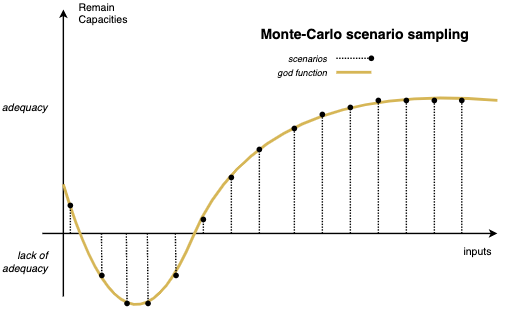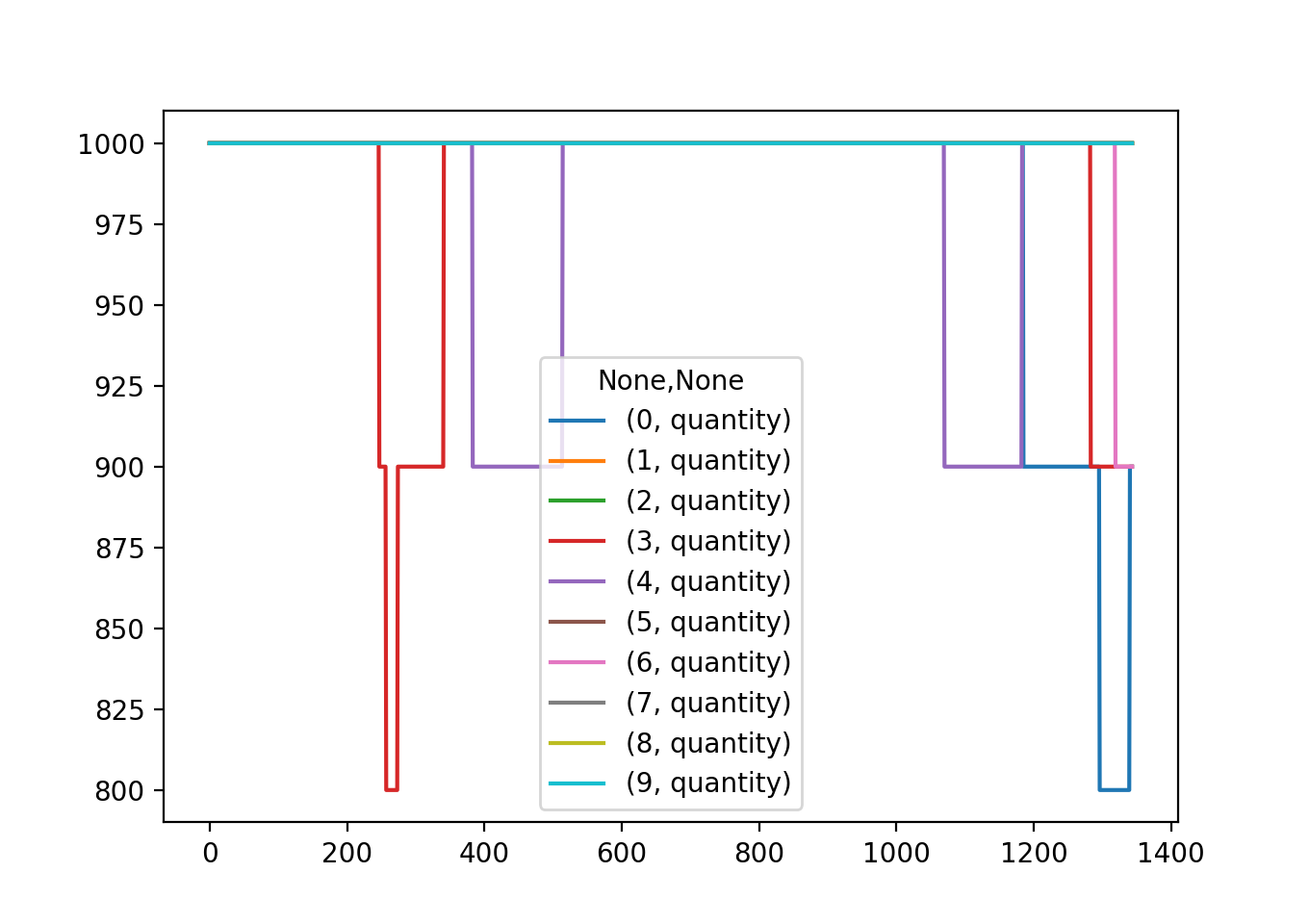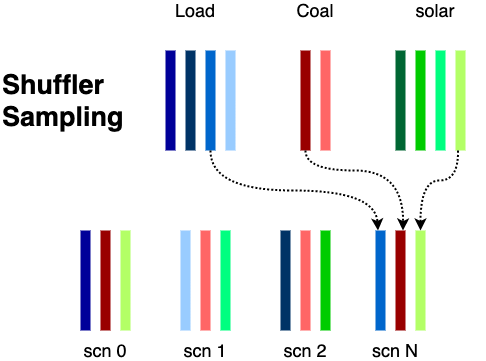# Workflow¶

## What is a stochastic study ?¶

Workflow is the preprocessing module for Hadar. It’s a toolbox to create pipelines to transform data for optimizer.

When you want to simulate a network adequacy, you can perform a deterministic computation. That means you believe you won’t have too much fluky behavior in the future. If you perform adequacy for the next hour or day, it’s a good hypothesis. But if you simulate network for the next week, month or year, it’s sound curious.

Are you sur wind will blow next week or sun will shines ? If not, you eolian or solar production could change. Can you warrant that no failure will occur on your network next month or next year ?

Of course, we can not predict future with such precision. It’s why we use stochastic computation. Stochastic means there are fluky behavior in the physics we want simulate. Simulation is quiet useless, if result is a unique result.

The best solution could be to compute a God function which tell you for each input variation (solar production, line, consumptions) what is the adequacy result. Like that, Hadar has just to analyze function, its derivatives, min, max, etc to predict future. But this God function doesn’t exist, we just have an algorithm which tell us adequacy according to one fixed set of input data.

It’s why we use Monte Carlo algorithm. Monte Carlo run many scenarios to analyze many different behavior. Scenario with more consumption in cities, less solar production, less coal production or one line deleted due to crash. By this method we recreate God function by sampling it with the Monte-Carlo method.Workflow will help user to generate these scenarios and sample them to create a stochastic study.

The main issue when we want to help people generating their scenarios is they are as many generating process as user. Therefore workflow is build upon a Stage and Pipeline Architecture.

## Stages, Pipelines & Plug¶

Stage is an atomic process applied on data. In workflow, data is a pandas Dataframe. Index is time. First column level is for scenario, second is for data (it could be anything like mean, max, sigma, …). Dataframe is represented below:

 scn 1 scn n … t mean max min … mean max min … 0 10 20 2 … 15 22 8 … 1 12 20 2 … 14 22 8 … … … … … … … … … …

A stage will perform compute to this Dataframe. As you assume it, stages can be linked together to create pipeline. Hadar has its own stages very generic, each user can build these stages and create these pipelines.

For examples, you have many coal production. Each production plan has 10 generators of 100 MW. That means a coal plan production has 1,000 MW of power. You know that sometime, some generators crash or need shutdown for maintenance. With Hadar you can create a pipeline to generate these fault scenarios.

# In this example, one timestep = one hour
import numpy as np
import matplotlib.pyplot as plt

# coal production over 8 weeks with hourly step
coal = pd.DataFrame({'quantity': np.ones(8 * 168) * 1000})

# Copy scenarios ten times
copy = hd.RepeatScenario(n=10)

# Apply on each scenario random fault, such as power drop is 100 MW, there is 0.1% chance of failure each hour
# if failure, it's a least for the whole day and until next week.
fault = hd.Fault(loss=100, occur_freq=0.001, downtime_min=24, downtime_max=168)

pipe = copy + fault
out = pipe.compute(coal)

out.plot()
plt.show()


Output:### Create its own Stage¶

RepeatScenario, Fault and all other are build upon Stage abstract class. A Stage is specified by its Plug (we will see sooner) and a _process_timeline(self, timeline: pd.DataFrame) -> pd.DataFrame to implement. timeline variable inside method is the data passed thought pipeline to transform.

For example, you need to multiply by 2 during your pipeline. You can create your stage by

class Twice(Stage):
def __init__(self):
Stage.__init__(self, FreePlug())

def _process_timeline(self, timelines: pd.DataFrame) -> pd.DataFrame:
return timelines * 2


Implement Stage will work every time. Often, you want to apply function independently for each scenario. You can of course handle yourself this mechanism to split current timeline apply method and rebuild at the end. Or use FocusStage, same thing but already coded. In this case, you need to inherent from FocusStage and implement _process_scenarios(self, n_scn: int, scenario: pd.DataFrame) -> pd.DataFrame method.

For example, you have thousand of scenarios, your stage has to generate gaussian series according to mean and sigma given.

class Gaussian(FocusStage):
def __init__(self):
FocusStage.__init__(self, plug=RestrictedPlug(input=['mean', 'sigma'], output=['gaussian']))

def _process_scenarios(self, n_scn: int, scenario: pd.DataFrame) -> pd.DataFrame:
scenario['gaussian'] = np.random.randn(scenario.shape)
scenario['gaussian'] *= scenario['sigma']
scenario['gaussian'] += scenario['mean']

return scenario.drop(['mean', 'sigma'], axis=1)


### What’s Plug ?¶

You are already see FreePlug and RestrictedPlug, what’s it ?

Stage are linked together to build pipeline. Some Stage accept every thing as input, like Twice, but other need specific data like Gaussian. How we know that stage can be link together and data given at the beginning of pipeline is correct for all pipeline.

First solution is saying : We don’t care about. During execution, if data is missing, error will be raised and it’s enough. Indeed… That’s work, but if pipeline job is heavy, takes hour, and failed just due to a misspelling column name, it’s ugly.

Plug object describe linkable constraint for Stage and Pipeline. Like Stage, Plug can be added together. In this case, constraint are merged. You can use FreePlug telling this Stage is not constraint and doesn’t expected any column name to run. Or use RestrictedPlug(inputs=[], outputs=[]) to specify inputs mandatory columns and new columns generated.

Plug arithmetic rules are described below ($$\emptyset$$ = FreePlug)

$\begin{split}\begin{array}{rcl} \emptyset & + & \emptyset & = & \emptyset \\ [a \rightarrow \alpha ] & + & \emptyset & = & [a \rightarrow \alpha ] \\ [a \rightarrow \alpha ] & + & [\alpha \rightarrow A]& = & [a \rightarrow A] \\ [a \rightarrow \alpha, \beta ] & + & [\alpha \rightarrow A]& = & [a \rightarrow A, \beta] \\ \end{array}\end{split}$

## Shuffler¶

User can create as many pipeline as he want. At the end, he could have some pipelines and input data or directly input data pre-generated. He needs to sampling this dataset to create study. For example, he could have 10 coal generation, 25 solar, 10 consumptions. He needs to create study with 100 scenarios.

Of course he can develop sampling algorithm, but he can also use Shuffler. Indeed Shuffler does a bit more than just sampling:

1. It is like a sink where user put pipeline or raw data. Shuffler will homogeneous them to create scenarios. Behind code, we use Timeline and PipelineTimeline class to homogenize data according to raw data or data from output pipeline.

2. It will schedule pipelines compute. If shuffler is used with pipeline, it will distribute pipeline running over computer cores. A good tips !

3. It samples data to create study scenarios.Below an example how to use Shuffler

shuffler = Shuffler()
# Add raw data as a numpy array
shuffler.add_data(name='solar', data=np.array([[1, 2, 3], [5, 6, 7]]))

# Add pipeline and its input data
i = pd.DataFrame({(0, 'a'): [3, 4, 5], (1, 'a'): [7, 8, 9]})
pipe = RepeatScenario(2) + ToShuffler('a')